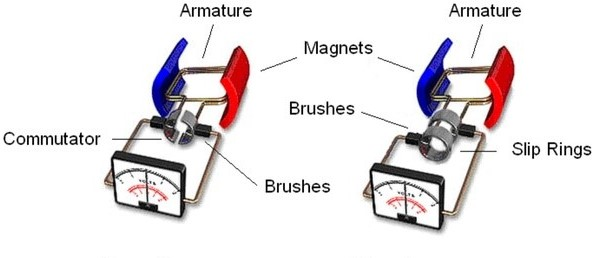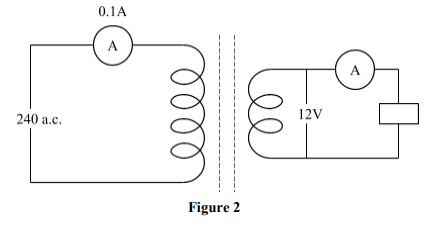• SECTION 01

(a)(i) Cork screw rule
If we consider ourselves driving a corkscrew in the direction of the current, then the direction of the corkscrew is the direction of the magnetic field(ii) Dynamo rule
Fleming's right-hand rule or dynamo rule shows the direction of induced current when a conductor attached to a circuit moves in a magnetic field.If the right hand is held with the thumb, index finger and middle finger mutually perpendicular to each other (at right angles) as shown in the diagram then, The thumb is pointed in the direction of the motion of the conductor relative to the magnetic field. The first finger is pointed in the direction of the magnetic fieldThen the second finger represents the direction of the induced or generated current within the conductor (from the terminal with lower electric potential to the terminal with higher electric potential, as in a voltage source)

• SECTION 02

(b)(i) Give one structural difference between A.C. and D.C. generators.
A.C generators use slip rings to transfer the generated current while D.C generator uses commutator to produce a dc current.AC and DC Generators

(ii) Mention one application of induction coil.
Induction coil is used to produce sparks which used to turn on or ignite petrol enginesInduction coil circuit diagram

• SECTION 03

(c) Figure 2 shows a transformer used to step down power. Assuming that there are no power losses, What will be the ammeter reading on the output part.Data and solution:

Assuming power is conserved, therefor Input Power = Output Power, Pp = P

But power, P = VI hence VpIp= VsIs

240 V x 0.1A = 12V x Is

I= (240V x 0.1A)/12V = 2A